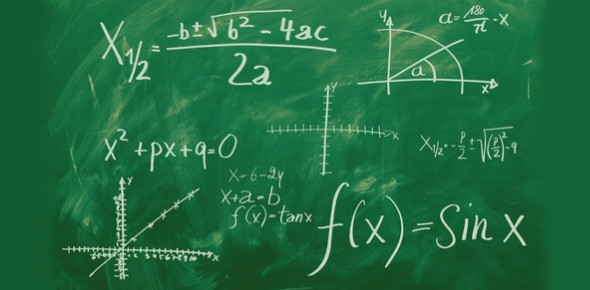# Algebraic Expressions Class 7 MCQ Quiz

10 Questions | Attempts: 5070
ShareSettingsThis is a practice test MCQ quiz for class 7 students to test their problem-solving skills, knowledge, and confidence on the topic of algebraic expressions. So, fellas, are you ready to assess yourself with this amazing quiz? If yes, take the quiz then. Variable expressions are sometimes quite tough to solve, but if your basics are clear, then you can easily solve any type of question. This quiz will help you to practice more in this topic, and then you'll score good marks in your exams too. Good luck!

• 1.
Write the expression for a) 32 plus a number n b) 58 less than a number n
• A.

32 + n, 58-n

• B.

32-n, 58+n

• C.

32-58+n

• D.

32-n+58

• 2.
The coefficient of x² in – 5x²y is
• A.

Y

• B.

-5

• C.

-5y

• D.

5y

• 3.
The value of 3x² – 5x + 3, if x=1 is
• A.

1

• B.

-1

• C.

0

• D.

2

• 4.
Solve the equation: –z² + 22z² – 5z – 11z² + 5z
• A.

10 z2

• B.

Z

• C.

-10 z2

• D.

Z

• 5.
The expression 4p²q – 4pq² + 1 is a
• A.

Binomial

• B.

Trinomial

• C.

Monomial

• D.

None of the above

• 6.
I= PRT so what does R=
• A.

I/PT

• B.

PT/I

• C.

T/PI

• D.

P/TI

• 7.
Which of the following pairs of terms is of unlike terms?
• A.

-p²q², 12p²q²

• B.

Qp², 13p²q

• C.

41,100

• D.

– 4x²; – Axy²

• 8.
(5x – y + 8) - (5x + 3y)
• A.

4x – 8

• B.

4y – 8

• C.

8 – 4y

• D.

4x + 8

• 9.
Subtracting -3x² – 1 from 2x + 1
• A.

– 3x² + 2x – 2

• B.

– 3x² + 2

• C.

3x² – 2

• D.

3x² + 2x + 2

• 10.
What is the coefficient of y2 in the expression 3y2 + 4x?
• A.

3

• B.

4

• C.

1

• D.

X

## Related TopicsBack to top
×

Wait!
Here's an interesting quiz for you.